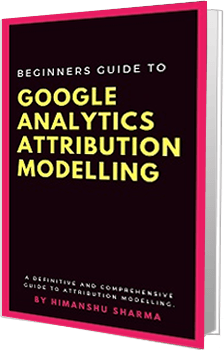The linear attribution model assigns equal credit for a conversion to each interaction on a conversion path

If you have a business model where each interaction is equally important for your conversions then you can use the linear interaction model.

For example, if you run a customer support service then each interaction with your customers is equally important for you. In that case, you can use the linear model.

The following examples will help you to understand how the conversion credit is calculated in the case of the linear attribution model:

Consider the following conversion path with a path length of four:

This conversion path can also be represented by the following data table:

Under the linear attribution model, the conversion credit for each interaction would be calculated as 1 / conversion path length.

Since the length of our conversion path is 4, the conversion credit for each interaction would be calculated as 1 / 4 = 25%:

• Campaign A gets 25% credit for the conversion.
• Campaign B gets 50% (25% + 25%) credit for the conversion.
• Campaign C gets 25% credit for the conversion.
`Get the E-book (52 Pages)`

Consider the second conversion path with a path length of three:

This conversion path can also be represented by the following data table:

Under the linear attribution model, the conversion credit for each interaction would be calculated as 1 / conversion path length.

Since the length of our conversion path is 3, the conversion credit for each interaction would be calculated as 1 / 3 = 33.33%

• Campaign A gets 66.66% (33.33%+33.33%) credit for the conversion.
• Campaign B gets 33.33% credit for the conversion.
• Campaign C gets 0% credit for the conversion (as campaign C is not on the conversion path).

The total conversion credit for campaign A so far

= (conversion credit for campaign A on the first conversion path + conversion credit for campaign A on the second conversion path) / 2

= (25% + 66.66%) / 2

= 45.83%

Total conversion credit for campaign B so far

= (conversion credit for campaign B on the first conversion path + conversion credit for campaign B on the second conversion path) / 2

= (50% + 33.33%) / 2

= 41.67%

Total conversion credit for campaign C so far

= (conversion credit for campaign C on the first conversion path + conversion credit for campaign C on the second conversion path) / 2

= (25% + 0%) / 2

= 12.5%

Consider the third conversion path with a path length of two:

This conversion path can also be represented by the following data table:

Since the length of our conversion path is two, the conversion credit for each interaction would be calculated as 1 / 2 = 50%

• Campaign A gets 50% credit for the conversion.
• Campaign B gets 50% credit for the conversion.
• Campaign C gets 0% credit for the conversion (as campaign C is not on the conversion path).

The total conversion credit for campaign A so far

= (conversion credit for campaign A on the first conversion path + conversion credit for campaign A on the second conversion path + conversion credit for campaign A on the third conversion path) / 3

= (25% + 66.66% + 50%) / 3

= 47.22%

The total conversion credit for campaign B so far

= (conversion credit for campaign B on the first conversion path + conversion credit for campaign B on the second conversion path + conversion credit for campaign B on the third conversion path) / 3

= (50% + 33.33% + 50%) / 3

= 44.44%

The total conversion credit for campaign C so far

= (conversion credit for campaign C on the first conversion path + conversion credit for campaign C on the second conversion path + conversion credit for campaign C on the third conversion path) / 3

= (25% + 0% + 0%) / 3

= 8.33%

If we assume that there are only three conversion paths in our selected time period, then the sum of conversion credit for campaigns A, B and C under the linear attribution model would be:  47.22% + 44.44% + 8.33% = 99.99% (rounded off to 100%)

Since the sum of the conversion credit for three campaigns is 100%, this proves that our conversion credit calculations are all correct.

## "How to use Digital Analytics to generate floods of new Sales and Customers without spending years figuring everything out on your own."

Here’s what we’re going to cover in this training…

#1 Why digital analytics is the key to online business success.

​#2 The number 1 reason why most marketers are not able to scale their advertising and maximize sales.

#4 ​Why you won’t get any competitive advantage in the marketplace just by knowing Google Analytics.

#5 The number 1 reason why conversion optimization is not working for your business.

​#7 How to learn and master digital analytics and conversion optimization in record time.

## My best selling books on Digital Analytics and Conversion Optimization

Maths and Stats for Web Analytics and Conversion Optimization
This expert guide will teach you how to leverage the knowledge of maths and statistics in order to accurately interpret data and take actions, which can quickly improve the bottom-line of your online business.

Master the Essentials of Email Marketing Analytics
This book focuses solely on the ‘analytics’ that power your email marketing optimization program and will help you dramatically reduce your cost per acquisition and increase marketing ROI by tracking the performance of the various KPIs and metrics used for email marketing.

Attribution modelling is the process of determining the most effective marketing channels for investment. This book has been written to help you implement attribution modelling. It will teach you how to leverage the knowledge of attribution modelling in order to allocate marketing budget and understand buying behaviour.